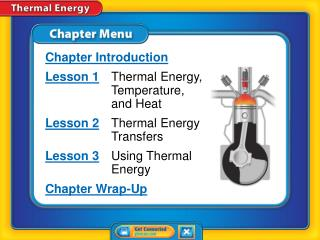Download PresentationChapter Menu

Loading in 2 Seconds...

# Chapter Menu - PowerPoint PPT Presentation

Chapter Introduction Lesson 1 Thermal Energy, Temperature, and Heat Lesson 2 Thermal Energy Transfers Lesson 3 Using Thermal Energy Chapter Wrap-Up. Chapter Menu. What do you think?.I am the owner, or an agent authorized to act on behalf of the owner, of the copyrighted work described.
Download Presentation## Chapter Menu

An Image/Link below is provided (as is) to download presentation

Download Policy: Content on the Website is provided to you AS IS for your information and personal use and may not be sold / licensed / shared on other websites without getting consent from its author.While downloading, if for some reason you are not able to download a presentation, the publisher may have deleted the file from their server.

- - - - - - - - - - - - - - - - - - - - - - - - - - E N D - - - - - - - - - - - - - - - - - - - - - - - - - -
Presentation Transcript
1. Chapter Introduction Lesson 1 Thermal Energy, Temperature, and Heat Lesson 2 Thermal Energy Transfers Lesson 3Using Thermal Energy Chapter Wrap-Up Chapter Menu

2. What do you think? Before you begin, decide if you agree or disagree with each of these statements. As you view this presentation, see if you change your mind about any of the statements. Chapter Introduction

3. 1. Temperature is the same as thermal energy. 2. Heat is the movement of thermal energy from a hotter object to a cooler object. 3. It takes a large amount of energy to significantly change the temperature of an object with a low specific heat. Do you agree or disagree? Chapter Introduction

4. 4. The thermal energy of an object can never be increased or decreased. 5. Car engines create energy. 6. Refrigerators cool food by moving thermal energy from inside the refrigerator to the outside. Do you agree or disagree? Chapter Introduction

5. Thermal Energy, Temperature, and Heat • How are temperature and kinetic energy related? • How do heat and thermal energy differ? Lesson 1 Reading Guide - KC

6. Kinetic and Potential Energy • Potential energy is stored energy due to the interaction between two objects. • Kinetic energy is the energy objects have due to motion. • Equation: • The potential energy plus the kinetic energy of an object is the mechanical energy of the object. Lesson 1-1

7. All matter made of small particles Particles of matter in motion Collisions between particles transmit energy Faster motion has more kinetic energy Temperature is average kinetic energy of the particles of a substance SI units for temperature are Kelvins (K) Kinetic Theory of Matter Kinetic Theory of Matter

8. What is thermal energy?(cont.) • The greater the average distance between particles, the greater the potential energy of the particles. • Thermal energy is the sum of the kinetic energy and the potential energy of the particles that make up a material. Lesson 1-2

9. What is temperature? • Temperaturerepresents the average kinetic energy of the particles that make up a material. Lesson 1-3

10. What is temperature?(cont.) • The greater the average kinetic energy of particles, the greater the temperature. • The particles in warmer air move at a greater average speed than the particles in colder air. Lesson 1-3

11. What is temperature?(cont.) • Thermometers are used to measure temperature. • Common temperature scales are Celsius (°C), Kelvin (K), and Fahrenheit (°F). Lesson 1-3

12. What is heat? • The movement of thermal energy from a warmer object to a cooler object is called heat. • All objects have thermal energy; however, you heat something when thermal energy transfers from one object to another. • The rate at which heating occurs depends on the difference in temperatures between the objects. Lesson 1-4

13. The greater the distance between two particles or two objects, the greater the potential energy. • Heat is the movement of thermal energy from a warmer object to a cooler object. Lesson 1 - VS

14. When thermal energy moves between a material and its environment, the material’s temperature changes. Lesson 1 - VS

15. Because particles are in motion, what type of energy do they have? A. thermal energy B. potential energy C. mechanical energy D. kinetic energy Lesson 1 – LR1

16. Particles that make up liquid and solid water have different potential energies, and therefore also have which of the following? A. different thermal energies B. different temperatures C. different kinetic energies D. the same thermal energy Lesson 1 – LR2

17. Which term refers to the average kinetic energy of the particles that make up a material? A. heat B. temperature C. potential energy D. thermal energy Lesson 1 – LR3

18. 1. Temperature is the same as thermal energy. 2. Heat is the movement of thermal energy from a hotter object to a cooler object. Do you agree or disagree? Lesson 1 - Now